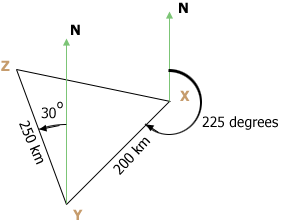SEARCH HOMEMath Central Quandaries & QueriesQuestion from olawale, a student: an airplane flew from a city X on a bearing of 225deg. to another city of Y a distance 200km away. it then flew from city Y on a bearing of 30deg.w to city Z a distance of 250km. calculate the distance from X to Z correct to the nearest whole number. and also calculate the bearing of city X from Z.Hi,

I assumed that the bearing of 255deg was measured clockwise from north and the bearing of 30deg.w was measured as 30 degrees west of north. This then is my diagram.You need to find the distance from X to Z and the bearing of X from Z.

In the triangle XZY you know the lengths of ZY and YX and from the information given you can find the measure of the angle ZYX. The Law of Cosines and the Law of Sines will information you need to solve the problem.

PennyMath Central is supported by the University of Regina and The Pacific Institute for the Mathematical Sciences.